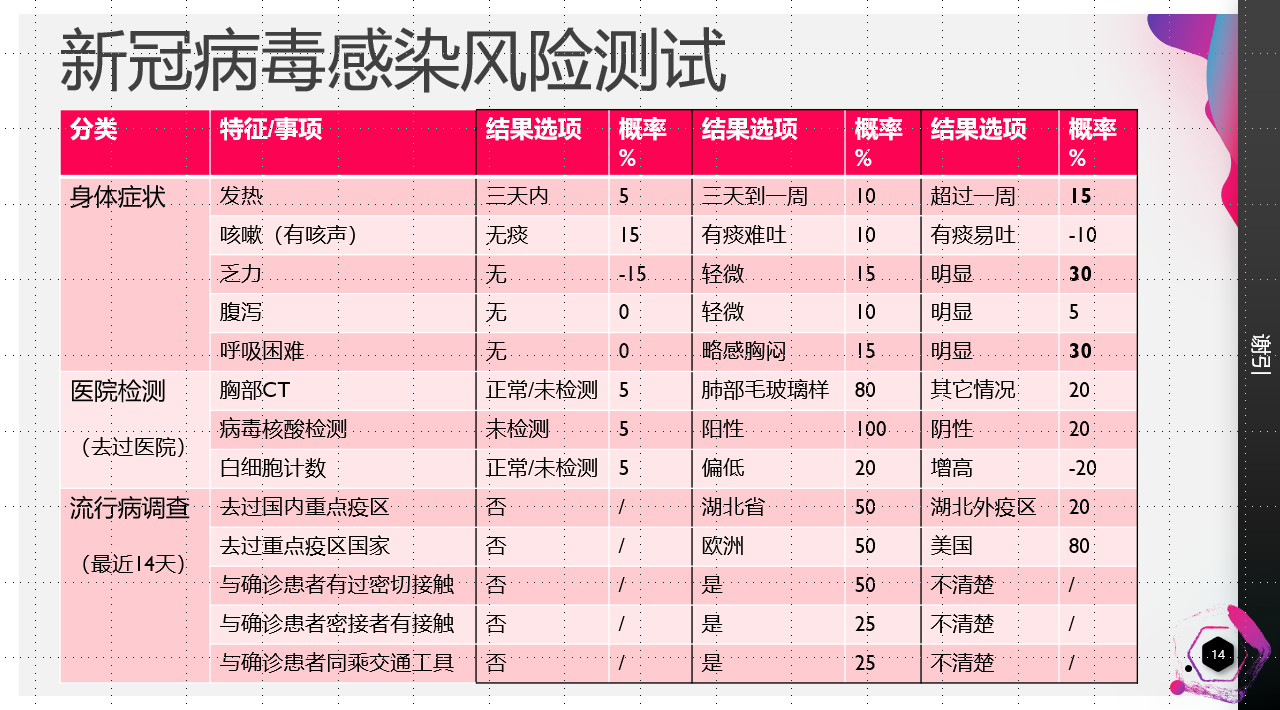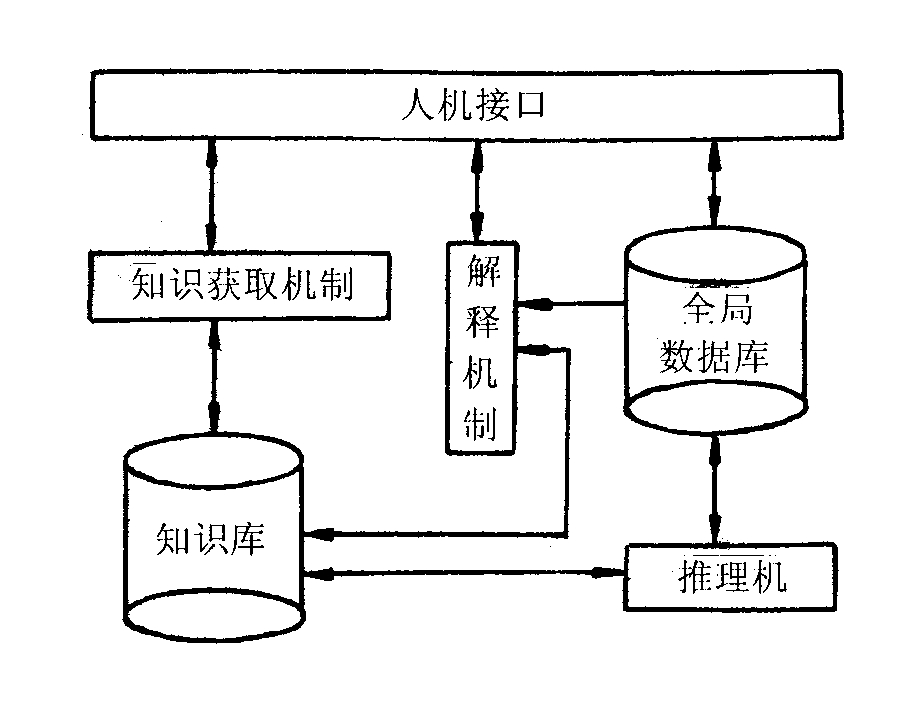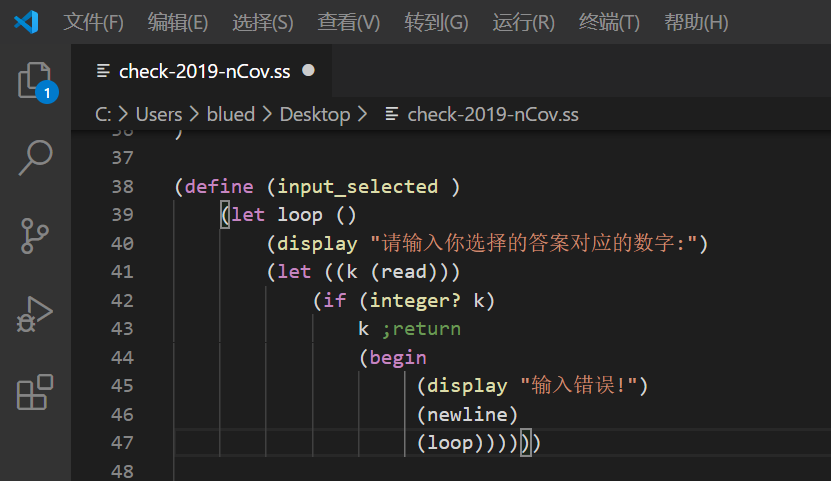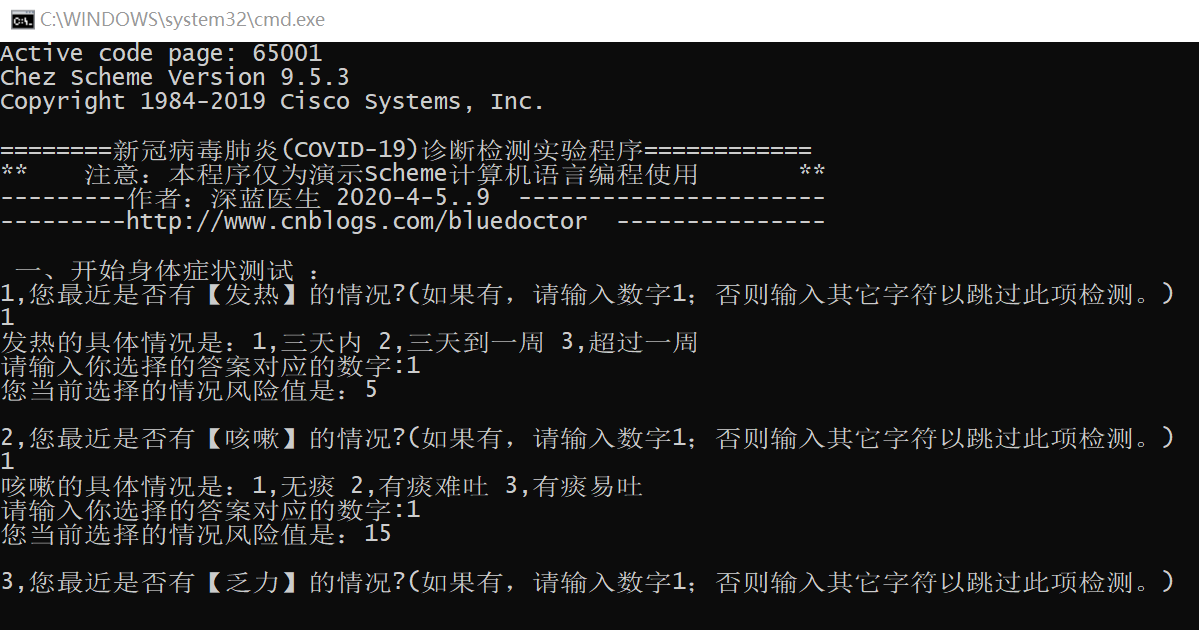## Scheme语言实例入门--怎样写一个“新型冠状病毒感染风险检测程序”

[原创文章，转载请阅读本文的结语--转载本文的申明]

# 小学生都能用的编程语言

2020的春季中小学受疫情影响，一直还没有开学，孩子宅在家说想做一个学校要求的研究项目，我就说你做一个怎么样通过编程来学习数学的小项目吧，用最简单的计算机语言来解决小学数学问题。虽然我是一个老码农，但一直不赞成教小学生学编程，觉得这是揠苗助长，小学生不应该过早的固化逻辑思维而放松形象思维，某些少儿编程机构居然教学C++游戏编程，我觉得这真是在摧残祖国的花朵。现在孩子宅在家 ，想让他学点什么好几次冒出学编程的想法都被自己给否决了，直到我看到数学老师要求同学们整理小学阶段的数学公式、概念，我看到有一个小朋友居然画出了平面几何体的“继承”关系，让我眼前一亮：这种抽象关系如果用程序来表示不正合适吗？明白抽象方法了，那么学编程问题就不大了。于是我在想应该教孩子学什么语言比较好：LOGO、VB还是炙手可热的Python？虽然我非常熟悉C#，但需要了解许多背景知识，还需要安装一个很大的框架环境，显然C#不适合小学生学习，Java也是。LOGO是老牌的儿童编程语言了，操控一个小海龟来画图很形象，VB入门简单，但要一个小学生熟悉它的集成开发环境要求还是高了点，选Python无非就是因为AI应用火它就火，除此之外我找不出它适合儿童使用的理由。

1，编程环境足够简单，一个命令行就行，不需要一个强大的IDE，否则用它还得熟悉很多菜单按钮和概念；

2，语法要足够简单，最好连变量都不需要定义，没有各种复杂的程序结构或语句，不需要了解方法、类、包、模块这些东西，拿来就能写程序跑起来；

3，数据结构要简单，什么数组、队列、堆栈或者树等等不要太多。

想到这里，唯一满足要求的就是Lisp语言了，它简单到只有3种最基本的数据结构：原子、表和字符串；只有一种语法，就是符号表达式，数据和函数都是采用符号表达式定义的，这种符号表达式称为S一表达式，它是原予和表的总称。Lisp衍生出了很多方言，形成一个庞大的Lisp语言家族，Scheme是其中最简单的方言，而且很长时间都是美国麻省理工学院计算机系的教学语言，Scheme的发明者和推动者都是数学家、科学家和教育学家，所以它一开始就有数学的基因，非常适合作为一种入门的计算机教学语言。前面说到孩子上数学课需要，那么Scheme语言就是不二之选了，B站有一个小学生用Scheme语言来表达三角形定理应用的小视频，可以点击这里查看

# 项目程序简介

既然决定教孩子Scheme语言，那我就得先熟悉一下它了，之前断断续续学习了几次，一直没有真正用过它所以始终没学好，现在疫情期间，正好可以用它来写一个程序练手，并且使用这个有实际意义的程序作为小学生学习Scheme语言的入门教程，于是有了本篇文章标题说的这个项目，一个2019新型冠状病毒肺炎（COVID-19）感染风险自助检测程序。为了更好的介绍Scheme语言，本文将结合这个实例程序来介绍Scheme语言的语法元素，于是有了这篇博客文章。读者可以先从下面的仓库地址克隆一份，包括源码和Scheme运行程序。有了这个风险测试表，如何简单有效的用程序表示，这也是我选择使用Scheme语言来写这个程序的原因，因为它的S表达式具有程序和数据的一致性，也就是说我们的知识数据可以表达为一种等价的程序结构，比如将上表的身体症状表达为下面的程序结构：

; 2019新型冠状病毒肺炎（COVID-19）感染风险自助检测程序
(define A1 (list "发热" (cons "三天内" 5) (cons "三天到一周" 10) (cons "超过一周" 15)))
(define A2 (list "咳嗽" (cons "无痰" 15) (cons "有痰难吐" 10) (cons "有痰易吐" -10)))
(define A3 (list "乏力" (cons "无" -15) (cons "轻微" 15) (cons "明显" 30)))
(define A4 (list "腹泻" (cons "无" 0) (cons "轻微" 10) (cons "明显" 5)))
(define A5 (list "呼吸困难" (cons "无" 0) (cons "略感胸闷" 15) (cons "明显" 30)))

# Scheme语言基础

## 1，表达式

### 符号类型(symbol)

> (quote a)
a
>'a
a

### 复合表达式

> (+ 1 2)
3

> '(+ 1 2)
(+ 1 2)

### S-表达式

Lisp 要求我们直接在抽象语法上工作。这个抽象的语法树用成对的括号表示。这样的结构在 Lisp 圈子里面被称为 sexp 表达式，俗称S-表达式。S-表达式的第一个单词决定了 sexp 的意义，剩下的单词都是参数，记住这一条规则足够了。这个规则使得我们不需要记忆其它编程语言那些复杂的语法结构，入门使用变得极其简单。我们在设计程序的时候应该始终围绕这个抽象语法进行，我们的程序设计的越抽象，那么程序就越接近问题的本质。

Lisp 程序看成是完全由"函数调用"这个单一的语法结构构成。 Lisp 里面没有为了算术表达式、或者逻辑表达式、或者语言的关键字，比如 IF 和 THEN，来准备特别的语法结构。所有的语言元素在 Lisp 里面都是按照这个简单一致的语法结构来安排，整个程序就是一个表达式，程序的运行就是对表达式进行求值。

## 2，原子

Lisp中有一个叫原子的东西，不可再分，是一个很基础的概念。原子可以是任何数，分数，小数，自然数，负数等等。原子可以是一个字母排列，当然其中可以夹杂数字和符号。除了表和所有函数以外均是原子。

Scheme没有直接说原子这个概念，但Scheme作为Lisp的方言，在形式上还是有原子这样的东西。所有的 Lisp/Scheme 表达式，要么是 1 这样的数原子，要么是包在括号里，由零个或多个表达式所构成的列表。所以可以这样说，List程序里面就是原子和表。这就是Lisp 表示法一个美丽的地方是：它是如此的简单！

## 3，表（list）

表是由多个相同或不同的数据连续组成的数据类型，它是编程中最常用的复合数据类型之一，很多过程操作都与它相关。下面是在Scheme中表的定义和相关操作：

> (define la (list 1 2 3 4 ))
>la
(1 2 3 4)
> (length la) ; 取得列表的长度
4
> (list-ref la 3) ; 取得列表第3项的值（从0开始）
4
> (list-set! la 2 99) ; 设定列表第2项的值为99
99
> la
(1 2 99 4)
> (define y (make-list 5 6)) ;创建列表
> y
(6 6 6 6 6)

> (define la (list 1 2 3 4 ))
>la
(1 2 3 4)
> (define la '(1 2 3 4 ))
>la
(1 2 3 4)

(define A1 (list "发热" (cons "三天内" 5) (cons "三天到一周" 10) (cons "超过一周" 15)))

## 4，点对(pair)

List/Scheme 的基本构成元素是所谓的 Pair。什么是 Pair 呢？？我们可以按照这个英语单词在日常生活中最常见的意思，比如一个坐标点，来想象这个最基础的数据结构。比如坐标（1，2）是一个点对。一个点对包含两个指针，每个指针指向一个值。我们用函数cons构造点对。比如说(cons 1 2)就构造出点对(1 . 2)。因为点对总是由函数cons构造，点对又叫做cons cell。点对左边的值可以用函数car取出来，右边的值可以由函数cdr取出来。比如列表(1 2 3 4)，实际上由点对构成：(1 . (2 . (3 . 4. ‘())。可以看出，列表本质是单向链表。

( 三天内 . 5  ) ( 三天到一周 . 10  )  (超过一周 . 15)

(cons "三天内" 5)(cons "三天到一周" 10)(cons "超过一周" 15)

>(car A1)

>(cdr A1)
( 三天内 . 5  ) ( 三天到一周 . 10  )  (超过一周 . 15)

## 5，向量(vector)

VECTOR表示为： #(1 2 3 4)

>(define v (vector 1 2 3 4 5))
#(1 2 3 4 5)
>(define v ‘#(1 2 3 4 5))
#(1 2 3 4 5)
> (vector-ref v 0) ; 求第n个变量的值
1
> (vector-length v) ; 求vector的长度
5
> (vector-set! v 2 "abc") ; 设定vector第n个元素的值
> v
#(1 2 "abc" 4 5)
> (define x (make-vector 5 6)) ; 创建向量表
> x
#(6 6 6 6 6)

(define QA (vector A1 A2 A3 A4 A5))

## 6，变量

(define 变量名 值)

> (let ((x 1) (y 2))
(+ x y))
3

let表达式的形式定义是：

(let [binds]

bodys

)

[binds] → ((p1 v1) (p2 v2) ...)

(set! 变量名 值)

(define Total_Risk 0) ;定义全局 风险总值 变量
;其它代码略
(display "单纯性发热，调低风险值。")(newline)
(set! Total_Risk (- Total_Risk 15))

Scheme语言是一种高级语言，和很多高级语言(如python，perl)一样，它的变量类型不是固定的，可以随时改变。

## 7，函数

Scheme语言中函数不同于其它语言的函数，它被称为过程（Procedure）。过程是一种数据类型，这也是为什么Scheme语言将程序和数据作为同一对象处理的原因。

> +
#<procedure +>

define不仅可以定义变量，还可以定义过程，因在Scheme语言中过程（或函数）都是一种数据类型，所以都可以通过define来定义。不同的是标准的过程定义要使用lambda这一关键字来标识。

> (define add1 (lambda (x) (+ x 1)))
6

(define (过程名 参数) (过程内容 …))

(define (input_selected )
(let loop ()
(display "请输入你选择的答案对应的数字:")
(if (integer? k)
k ;return
(begin
(display "输入错误!")
(newline)
(loop))))))

## 8，类型判断

Scheme语言中所有判断都是用类型名加问号再加相应的常量或变量构成，例如上面定义的函数input_selected 中使用 integer? 来判断用户输入的内容是否是一个整数：(integer? k)

(null? (cdr name-values))

## 9，逻辑运算

 ;测试是否有严重腹泻并且没有咳嗽
(let ((FU_XIE (have_attribute_inResult Ctx_Attributes "腹泻")))
(if (and    (not (null? FU_XIE))
(equal? "明显" (car (cdr FU_XIE)))
(null? KE_SOU)
)
(set! Total_Risk 0) ;为严重腹泻引起的乏力,不会是新冠感染。
(begin
(display "乏力伴随咳嗽或者发热，调高风险值。")(newline)
(set! Total_Risk (* Total_Risk 2))
)
)
)

and和or表达式都可以处理多个逻辑表达式参数，例如上面的and 表达式处理了三个逻辑判断表达式。

## 10，代码块(form)

块(form)是Scheme语言中的最小程序单元，一个Scheme语言程序是由一个或多个form构成。没有特殊说明的情况下 form 都由小括号括起来，所以一个form最小可以是一个表达式，也可以是一个变量定义，一个过程定义。form允许嵌套，这使它可以轻松的实现复杂的表达式，同时也是一种非常有自己特色的表达式。有时候需要将多个表达式组合成一个form，比如在分支判断后执行多个操作，需要在一个块中，这个功能可以通过begin表达式来实现，例如上面逻辑运算的例子。

## 11，分支结构

### if结构：

Scheme语言的if结构有两种格式：
(if (测试条件）(满足测试条件时 要执行的过程1))
(if (测试条件）(满足测试条件时 要执行的过程1)
(否则 要执行的过程2) )

### cond结构：

Scheme语言中的cond结构类似于C语言中的switch结构，cond的格式为：

(cond ((测试) 操作) … (else 操作))

cond结构的每个测试都可以是不同的逻辑表达式，相当于多个嵌套的if...else if...结构，在当前实例项目中就曾经使用cond结构来优化，示例代码如下:

;从结果中判断是否有指定的症状属性；如果有，返回症状特征表；如果没有，返回空表
(define (have_attribute_inResult result attName)
(let loop ( (lst result) )
(let ( (car_lst (car lst))  (cdr_lst (cdr lst)))
;(if (equal? attName (car car_lst))
;    car_lst
;     (if (null? cdr_lst)
;        '()
;        (loop cdr_lst)
;    )
;)
;
(cond   ((equal? attName (car car_lst))  car_lst )
((null? cdr_lst) '())
(else (loop cdr_lst))
)
)
)
) 

### case结构：

case结构和cond结构有点类似，它的格式为：

(case (表达式) ((值) 操作)) ... (else 操作)))

case结构中的值可以是复合类型数据，如列表，向量表等，只要列表中含有表达式的这个结果，则进行相应的操作，如下面的代码：

 >(case (* 2 3)
((2 3 5 7) 'prime)
((1 4 6 8 9) 'composite))
composite

(display "2）最近14天，您是否去过 重点疫区国家？（0-未去过，1-意大利、西班牙，2-欧洲其它地方，3-美国，4-世界其它地方）")
(newline)
(set! Total_Risk (+ Total_Risk
(case (input_selected)
((0) 0)
((1) 50)
((2 4) 30)
((3) 80)
(else 0)
)
))

## 12，循环结构

### 使用递归实现循环：

Scheme最原始的语法结构是没有循环的，要实现循环功能，可以通过递归调用过程实现：

>(define loop
(lambda(x y)
(if (<= x y)
(begin
(display x) (display #\space)
(set! x (+ x 1))
(loop x y)))))
>(loop 1 10)
1 2 3 4 5 6 7 8 9 10

### 使用命名let:

let表达式本质上是一个Scheme语法糖，它内部转换成了lambda表达式调用。命名let在Scheme中与尾递归有相似的效果，具体可以参考这篇文章

(define (input_selected )
(let loop ()
(display "请输入你选择的答案对应的数字:")
(if (integer? k)
k ;return
(begin
(display "输入错误!")
(newline)
(loop))))))

(define (have_attribute_inResult result attName)
(let loop ( (lst result) )
(let ( (car_lst (car lst))  (cdr_lst (cdr lst)))
(cond   ((equal? attName (car car_lst))  car_lst )
((null? cdr_lst) '())
(else (loop cdr_lst))
)
)
)
)

### 使用do循环表达式：

    (do binds
(predicate value)
body)

[binds] - ((p1 i1 u1) (p2 i2u2)...)

 1 ;获取症状特征列表指定的特征序号（从1开始的序号）对应的风险值
2 (define (get-index-value lst_attribute index)
3     (do ((name-values (cdr lst_attribute) (cdr name-values))
4          (i 1 (+ i 1)))
5         ( (or (= i index) (null? (cdr name-values))) ;break
6           (cdr (car name-values)) ;return value
7         )
8         ;(display (cdr name-values ))
9         ;(newline)
10     )
11 )

# 系统设计方案

## 2，专家系统• 人机界面：采用交互式命令行方式，系统提问，用户根据提是选择回答问题，引导用户完成所有问题的测试。
• 知识库：将“新冠病毒感染风险测试表”的知识使用Scheme程序表示。
• 全局数据库：一个存储当前患者对象诊断数据的“特征上下文”对象，它是一个列表。
• 解释机制：每当用户回答一个问题，都会给出当前特征项的风险值，并在诊断完成后给出诊断数据。
• 推理机：它包括2个工作，一是根据用户回答的问题计算感染风险的累加值，另一个是根据一些特殊的风险属性相关性规则，来修正风险值，比如对于乏力症状特征与其它症状特征之间的关系进行处理的规则。

## 3，推理机制

(list "发热" (cons "三天内" 5) (cons "三天到一周" 10) (cons "超过一周" 15))

(define A1 (list "发热" (cons "三天内" 5) (cons "三天到一周" 10) (cons "超过一周" 15)))

;定义 患者的身体症状特征
(define A1 (list "发热" (cons "三天内" 5) (cons "三天到一周" 10) (cons "超过一周" 15)))
(define A2 (list "咳嗽" (cons "无痰" 15) (cons "有痰难吐" 10) (cons "有痰易吐" -10)))
(define A3 (list "乏力" (cons "无" -15) (cons "轻微" 15) (cons "明显" 30)))
(define A4 (list "腹泻" (cons "无" 0) (cons "轻微" 10) (cons "明显" 5)))
(define A5 (list "呼吸困难" (cons "无" 0) (cons "略感胸闷" 15) (cons "明显" 30)))
(define QA (vector A1 A2 A3 A4 A5))

;定义患者的医院化验检查结果特征
(define B1 (list "胸部CT" (cons "正常/未检测" 1) (cons "肺部毛玻璃样" 80) (cons "其它情况" 20)))
(define B2 (list "病毒核酸检测" (cons "未检测" 5) (cons "阳性" 100) (cons "阴性" 20)))
(define B3 (list "白细胞计数" (cons "正常/未检测" 1) (cons "偏低" 20) (cons "增高" -20)))
(define QB (vector B1 B2 B3 ))

( (特征名1 特征值名 特质值) (特征名2 特征值名 特质值) ...)

 ((发热 三天内 5) (咳嗽 无痰 15) (乏力 无 -15) (腹泻 无 0) (呼吸困难 无 0))

(define Ctx_Attributes '())

## 4，推理机的实现

(define (process-question listAttributes)
(let loop ((i 0) (j (- (vector-length listAttributes) 1)))
(display (+ i 1))
(display ",您最近是否有【")
(let ((Ai (vector-ref listAttributes i)))
(display (car Ai))
(display "】的情况?(如果有，请输入数字1；否则输入其它字符以跳过此项检测。)")
(newline)
;(let ((input 1)) ;test
(let ((input (read)) (Ai_Name (car Ai)))
(if (and (integer? input) (= input 1))
(begin
(display Ai_Name)
(display "的具体情况是：")
(show-attribute Ai)
(newline)
(let ((q_index (input_selected )))
(display "您当前选择的情况风险值是：")
(let ((curr_risk (get-index-value Ai q_index)))
(display curr_risk)
(set! Ctx_Attributes (append Ctx_Attributes
(list (list Ai_Name (get-index-name Ai q_index) curr_risk ))))
(set! Total_Risk (+ Total_Risk curr_risk))
)

)
)
(begin
(display "您没有【")
(display Ai_Name)
(display "】的情况。")
)
)
)
(newline)
(newline)
)

(if (< i j)
(loop (+ i 1) j))
))

(display " 一、开始身体症状测试 ：")(newline)
(process-question QA)
(display "初步诊断详细内容：")
(display Ctx_Attributes )
(newline)
;其它代码略
(display " 二、开始进行【医院检测结果】分析 ：")(newline)
(process-question QB)

# 开发和运行# 结语

## 1，转载本文的申明

（本文转载自 深蓝医生 的博客文章，原文地址：https://www.cnblogs.com/bluedoctor/p/12669369.html

## 2，本项目程序使用版权须知

posted on 2020-04-11 23:03  深蓝医生  阅读(1947)  评论(4编辑  收藏  举报